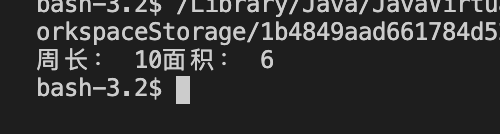• java代码-已知长方形的长和宽 求长方形周长面积代码
• Java初学者——求长方形的面积和周长。

千次阅读 多人点赞 2019-03-01 15:26:32
刚开始学java，给自己留点笔记。 所用编辑器是Eclipse。 面向过程的方法 package test; import java.util.*; public class Rectangle { public static void main(String[] args) { Scanner i...

注：本文非标准教程，仅是总结个人学习过程，可能存在纰漏，如有错误之处欢迎留言告知，非常感谢

刚开始学java，给自己留点笔记。

所用编辑器是Eclipse

面向过程的解法

package test;
import java.util.*;
public class Rectangle {

public static void main(String[] args) {
Scanner in=new Scanner(System.in);
System.out.println("请输入长方形的长和宽");
int a=in.nextInt();
int b=in.nextInt();
System.out.println("面积是："+(a*b));
System.out.println("周长是："+(2*(a+b)));

}

}

笼统的过程开发，就像用C做一样,学习java应该减少使用这种做法。

面向对象的解法1

package test;
import java.io.*;
import java.util.*;
public class Rectangle {
public int longth,width;
public int getArea(int l,int w) {
long=l;
width=w;
System.out.println("面积："+LongSK*Width);
return l*w;

}
public int getRrimeter(int l,int w) {
longth=l;
width=w;
System.out.println("周长："+2*(longth+width));
return 2*(l+w);
}

public static void main(String[] args) {
Scanner in=new Scanner(System.in);

System.out.println("请输入长方形的长和宽");
int l=in.nextInt();
int w=in.nextInt();
int A=new Rectangle().getArea(l, w);
A=new Rectangle().getPerimeter(l, w);
}

}

去请教了老师，然后老师说这个解法不是很好(哭）
原因是longth和width可以在方法里使用，方法不需要设置参数来代替longth和width，所以这个解法不好。

面向对象的解法2

package test;
import java.util.*;
public class Rectangle {

int longth,width;
public int getArea() {
//System.out.println("面积："+longth*width);
return longth*width;

}
public int getPerimeter() {
//System.out.println("周长："+2*(longth+width));
return 2*(longth+width);
}

public static void main(String[] args) {
Scanner in=new Scanner(System.in);
int B,C;
Rectangle A=new Rectangle();
System.out.println("请输入长方形的长和宽");
A.longth=in.nextInt();
A.width=in.nextInt();
B= A.getArea();
C=A.getPerimeter();
System.out.println("面积："+B);
System.out.println("周长："+C);

}

}

这个就不在方法里带参数。。

总结：

1、int A=new Rectangle().SetArea(l, w); 用main函数里的变量来调用类里的方法。

2、学习到了对于属性在方法里的使用。

(方法相当于主类里的函数；属性是主类里的定义的变量。)

3、import java.util.*; 是导入包，相当于C++里的 using namespace std;

展开全文• java实现一个长方形周长面积的计算,并用于子类正方形的相关计算
• Java面向对象的创建类-调用类完成的一个小程序，帮助更好理解。java
• 要求是：定义一个长方形类，定义 求周长面积的方法，然后定义了一个测试Test，进行测试。 执行代码 //定义一个长方形类，定义 求周长面积的方法，然后定义了一个测试Test，进行测试。 class Rectangle{ //长 ...

面向对象的练习题

要求是：定义一个长方形类，定义 求周长和面积的方法，然后定义了一个测试Test，进行测试。

执行代码

//定义一个长方形类，定义 求周长和面积的方法，然后定义了一个测试Test，进行测试。

class Rectangle{
//长
private int length;
//宽
private int width;
1
public Rectangle(int length,int width){
this.length = length;
this.width = width;
}

//求周长
public int getPerimeter(){
return (length + width) * 2;
}

//求面积
public int getArea(){
return length*width;
}
}

class Test{
public static void main(String[] args) {
Rectangle r = new Rectangle(2, 3);
System.out.println("周长： "+ r.getPerimeter()+"面积： "+ r.getArea());
}
}

运行结果展开全文• java代码-已知长方形的长和宽，求长方形周长面积代码
• (事实上前人就是已经把很多类和接口给我们编写好了，如Java的类库) //项目开始前，构建时就要确定模块，定义接口，比如就先定义这个例子中的接口，由甲来完成 //紧接着乙就可以开始编写实现类的代码了，同时丙也可以...

//面向的重要体现就是接口的使用 //甲 interface zfx { double mianji(); double zhchang(); } //乙 class zfxing implements zfx { private double Chang; private double kuan; zfxing(float i, float j){ this。

Chang=i; this。kuan=j; } public double mianji() { return Chang*kuan; } public double zhchang() { return 2*(Chang+kuan); } } //丙 class showout{ public void showmianji(zfx z) {//体现面向对象思想：方法依赖于接口类，而不是一个特定的类 System。

out。println(z。mianji());//如在实际项目中类的实现部分可能还没完成 System。out。println(z。zhchang());//你就可以先在这里先应用了。 } } //丁 class test{ public static void main(String[] args) { new showout()。

showmianji(new zfxing(12,25));//体现面向对象思想：模块的组装，项目快结束时， //我们只要传入能能实现这个接口的类作为参数，就能把大家各自编写的代码组合起来！ } //这里我们看到面向对象的程序设计是多么的巧妙，能分工协作，能发挥人多的优势！ } //假若有人已经把接口定义好并实现了这个接口，我们就省事了，我们就直接编写代码实例化类， //并用接口来向实现接口的类发送消息！(事实上前人就是已经把很多类和接口给我们编写好了，如Java的类库) //项目开始前，构建时就要确定模块，定义接口，比如就先定义这个例子中的接口，由甲来完成 //紧接着乙就可以开始编写实现类的代码了，同时丙也可以开始编写需要这个类的项目部分了 //最后，由丁就可以完成组装！希望你能得到一些帮助，希望你能因此对面向对象的思路有所理解。

全部

展开全文• 展开全部packagedemo;importjava.util.Scanner;publicclassTriangle{/***等腰*/publicstaticfinalintISOSCELES=1;/***等边*/publicstaticfinalintEQUILATERAL=2;/***直角32313133353236313431303231363533e...

展开全部

package demo;

import java.util.Scanner;

public class Triangle

{

/**

* 等腰

*/

public static final int ISOSCELES = 1;

/**

* 等边

*/

public static final int EQUILATERAL = 2;

/**

* 直角32313133353236313431303231363533e78988e69d8331333335343938

*/

public static final int RIGHTANGLE = 4;

/**

* 普通

*/

public static final int GENERAL = 6;

/**

* 不构成三角形

*/

public static final int NOTHING = 7;

double a;

double b;

double c;

public Triangle ( double a, double b, double c )

{

this.a = a;

this.b = b;

this.c = c;

}

public static int isWhichOne ( Triangle triangle )

{

double a = triangle.a;

double b = triangle.b;

double c = triangle.c;

if (a + b > c && a + c > b && b + c > a && Math.abs (a - b)

{

if (a == b && a == c)

{

return Triangle.EQUILATERAL | Triangle.ISOSCELES;

}

else if (a * a + b * b == c * c || a * a + c * c == b * b || c * c + b * b == a * a)

{

if (a == b || a == c || b == c)

{

return Triangle.RIGHTANGLE | Triangle.ISOSCELES;

}

return Triangle.RIGHTANGLE;

}

else if (a == b || a == c || b == c)

{

return Triangle.ISOSCELES;

}

else

{

return Triangle.GENERAL;

}

}

else

{

return Triangle.NOTHING;

}

}

public static double perimeter ( Triangle triangle )

{

return triangle.a + triangle.b + triangle.c;

}

public static double area ( Triangle triangle )

{

double a = triangle.a;

double b = triangle.b;

double c = triangle.c;

return 1.d / 4 * Math.sqrt (( a + b + c ) * ( a + b - c ) * ( a + c - b ) * ( b + c - a ));

}

public static void translate ( Triangle triangle )

{

int type = isWhichOne (triangle);

switch (type)

{

case Triangle.ISOSCELES:

System.out.println ("等腰三角形");

break;

case ( Triangle.EQUILATERAL | Triangle.ISOSCELES ):

System.out.println ("等边三角形 + 等腰三角形");

break;

case Triangle.GENERAL:

System.out.println ("普通三角形");

break;

case ( Triangle.RIGHTANGLE | Triangle.ISOSCELES ):

System.out.println ("直角三角形 + 等腰三角形");

break;

case Triangle.RIGHTANGLE:

System.out.println ("直角三角形");

break;

default:

System.out.println ("不构成三角形");

break;

}

if (type != Triangle.NOTHING)

{

System.out.println ("周长是：" + perimeter (triangle));

System.out.println ("面积是：" + area (triangle));

}

}

public static void main ( String[] args )

{

System.out.println ("输入构成三角形的三边长：(空格隔开)\n退出按 (n/N), 否则继续: ");

String error = "输入有误，请重新输入: ";

String reg = "^\\d+\\s+\\d+\\s+\\d+\$";

Scanner scanner = new Scanner (System.in);

while (scanner.hasNextLine ())

{

String line = scanner.nextLine ().trim ();

if ("n".equalsIgnoreCase (line))

{

break;

}

if (!line.matches (reg))

{

System.err.println (error);

}

else

{

try

{

String[] input = line.split ("\\s+");

int a = Integer.parseInt (input);

int b = Integer.parseInt (input);

int c = Integer.parseInt (input);

Triangle triangle = new Triangle (a, b, c);

translate (triangle);

}

catch (NumberFormatException e)

{

System.err.println (error);

}

System.out.println ("退出按 (n/N), 否则继续: ");

}

}

scanner.close ();

}

}

展开全文• java代码-已知长方形的长和宽，求长方形周长面积 26 张钧杰代码
• java代码-例2-2已知长方形的长和宽，求长方形周长面积代码
• Java—计算长方形周长面积（类和对象） Description 设计一个长方形类Rect，计算长方形周长面积。 成员变量：整型、私有的数据成员length(长)、width(宽)； 构造方法如下： （1）Rect(int length) —— 1个...
• java代码-定义并输出常量和变量 已经长方形的长和宽，求长方形周长面积代码
• 满意答案daikuanii推荐于 2017.11.23采纳率：50%等级：12已帮助：4607人//看看我这个程序把 比较符合面向对象的思想，告诉搂住一...import java.util.Scanner;public class Ex {public static int squ(int x,int y){...java求长方形面积
• 1.首先，我们定义一个... 求面积：长宽 class Rectangle { //长方形的长 private int length; //长方形的宽 private int width; public Rectangle(){} //这里只提供setXxx()，应为getXxx()在这暂时不用...JAVA
• java代码-已知长方形的长和宽,求长方形周长面积.代码
• 设计一个长方形类Rect，计算长方形周长面积。 成员变量：整型、私有的数据成员length(长)、width(宽)； 构造方法如下： （1）Rect(int length) —— 1个整数表示正方形的边长 （2）Rect...
• 设计一个长方形类Rect，计算长方形周长面积。 成员变量：整型、私有的数据成员length(长)、width(宽)； 构造方法如下： （1）Rect(int length) —— 1个整数表示正方形的边长 （2）Rect(int length, int ...
• java计算长方体面积和周长

千次阅读 2019-10-30 13:09:28
import java.util.Scanner; public class Yang1014 { public static void main(String[] args) { double width,height,area,girth; System.out.println(“请输入长方形的宽:”); width=new Scanner(System.in)....
• 定义一个长方形(Rectangle)类,定义求周长(length)和面积(area)的方法, 然后定义一个测试类Test，进行测试。 长方形类 public class Rectangle { private int wide; private int longg; public static int ...java 开发语言 后端
• 求矩形（长方形）的周长面积案例   package day07; import java.util.Scanner; public class Rectangle { /** * 需求： * 求一长方形周长面积 */ //定义成员变量，长和宽 private int length;...求长方形的周长
• 设计一个长方形类Rect，计算长方形周长面积。 成员变量：整型、私有的数据成员length(长)、width(宽)； 构造方法如下： （1）Rect(int length) —— 1个整数表示正方形的边长 （2）Rect(int length, int ...
• 定义一个长方形类， 长和宽是它的两个属性，定义为成员变量，求长方形面积周长。 1.代码实现 /* 定义一个长方形类， 长和宽是它的两个属性，定义为成员变量，求长方形面积周长 */ import java.util....java
• java定义一个方法计算三角形，长方形，圆形面积周长 /** * 定义一个方法，返回一个圆的周长 半径 * 定义一个方法，返回一个圆的面积 半径 * 定义一个方法，返回一个矩形的周长 长 宽 * 定义一个方法，返回一...java spring 反射 字符串 leetcode
• java代码-例2-2 已知长方形的长和宽，求长方形周长面积代码
• java：键盘录入长方形的长和宽，计算长方形周长面积，请使用面向对象的方式解决！ //测试类： public class Test { public static void main(String[] args){ MyMath calculation=new MyMath(); //加法 ...java 算法
• Java编写点线，三角形长方形圆形等面积周长** 初学者 设计一个能够描述点（point）、线(line)、圆（circle）、矩形（rectangle）和三角形（triangle）等几何形状的类体系，能够计算不同形状的周长面积（如果能够......

java长方形面积周长java 订阅